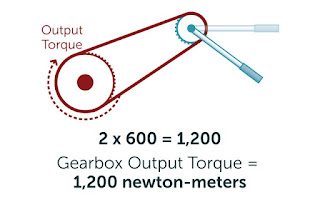# How to Calculate Gear Ratios and Torque

In the theory of mechanics, torque is a twisting force applied to an object. The force is applied by a lever which can be real or imaginary; the longer the lever or greater the force, the greater the torque. You express the units of torque as the length of the lever times force, with examples being foot-pounds or newton-meters.

Gears are useful for multiplying or dividing torque, whether the gears mesh directly or through a belt or chain; the ratio of the size of the gears determines whether they increase or decrease torque. To calculate gear ratios and the effect they have on torque, you need the size of each gear and the torque acting on the first gear, which mechanics call the “driver.”

Step 1Multiply the force acting on the first gear by the first gear's radius. If, for instance, a force of 4,000 newtons acts on the gear, and it measures 0.15 meters in radius, 4,000 x 0.15 = 600. The gear turns with 600 newton-meters of torque.

Step 2Divide the second gear's radius by the first gear's. If the second gear, for instance, measures 0.3 meters in radius: 0.3 / 0.15 = 2. The system's gear ratio is 2-to-1.Multiply the gear ratio by the input torque that you calculated in Step 1: 2 x 600 = 1,200 newton-meters. This is the gearbox's output torque.